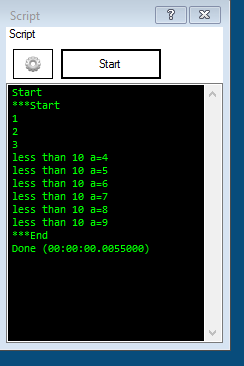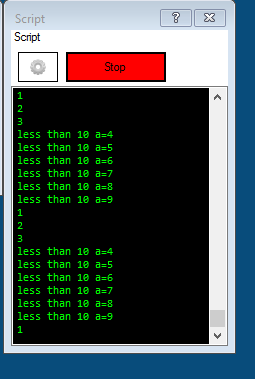#### Welcome to Synthiam!

Program robots using technologies created by industry experts. ARC is our free-to-use robot programming software that makes features like vision recognition, navigation, and artificial intelligence easy.

## Elseif Is Broken

@DJ,

The ElseIf is broken:

The code below never stops !

#### `Code:`

``\$buffer="[123 456 789]"\$len=Length(\$buffer)\$ix=0\$start=-1\$end=-1repeatwhile(\$ix<\$len)  \$ch=GetCharAt(\$buffer, \$ix)  if (\$ch="[")    print("found start")    \$start=\$ix  elseif (\$start>=0 AND \$ch="]")    print("found end")    \$end=\$ix    # break    \$ix=\$len+1  endif  print("increment ix")  \$ix=\$ix+1endrepeatwhileprint(\$ix)print(\$start)print(\$end)``

if you remove the ElseIf it works as expected.

`Code:\$buffer="[123 456 789]"\$len=Length(\$buffer)\$ix=0\$start=-1\$end=-1repeatwhile(\$ix<\$len) \$ch=GetCharAt(\$buffer, \$ix) if (\$ch="[") print("found start") \$start=\$ix endif if (\$start>=0 AND \$ch="]") print("found end") \$end=\$ix # break \$ix=\$len+1 endif print("increment ix") \$ix=\$ix+1endrepeatwhileprint(\$ix)print(\$start)print(\$end)`

This bug must be recent, i've used before the ElseIf, or did i miss something ?

ARC Early Access will give you immediate updates and new features needed to unleash your robot's potential!Related Content
Synthiam
Based on your post activity, we found some content that may be interesting to you. Explore these other tutorials and community conversations.
#4
Hi @PTP
I tried the simplest

If
ElseIF
EndIf

I could think of and this is working both trying \$a=1 and \$a=2

`Code:\$a=2:loopif (\$a=1) Print( "\$a= " + \$a) elseif (\$a=2) Print( "\$a= " + \$a)endif Sleep( 2000 ) Goto(loop)`

I'm using ARC 2017.05.14

Maybe the ElseIf issue has been fixed

Regards,
Frank
PRO
USA
#5
Frank:

Did you tried my code and is working for you ?
I just tried my code with the new version and the bug is still present.

Simple example 2 (broken):

`Code:\$a=11print("Start")if (\$a=1) print("one")elseif (\$a=3) print("three")elseif (\$a=5) print("five")endifprint("End")`

output:
script does not stop and keeps printing "Start".

Simple example 3 (broken) WORKING:

`Code:\$a=11print("***Start")if (\$a=1) print("one")ELSEif (\$a=3) print("three")ELSEif (\$a=5) print("five")ELSE print("another number")endifprint("End")`

output:
Start
***Start
another number
End

#EDIT: This example works, Start is not duplicated, i confused the second start with ARC Start
#6
Nevermind
PRO
USA
#7
Frank:

didn't took me long to break your code`Code:\$a=3:loopprint("before")if (\$a=1) Print( "\$a= " + \$a)ELSEif (\$a=2) Print( "\$a= " + \$a)endifprint("after")Sleep( 2000 )Goto(loop)`

The code above is broken
#8
I can confirm what @ptp is saying... None of his examples seem to work...
PRO
USA
#9
@Richard R:
Last version ?
#10
#11
I'll take a look
#13
`Code:\$a=11print("Start")if (\$a=1) print("one")ELSEif (\$a=3) print("three")ELSEif (\$a=5) print("five")ELSE print("another number")endifprint("End")`

Outputs (correct):

#### Quote:

Start
Start
another number
End
Done (00:00:00.0156256)

----------------------------------------------------------------------------------------------

`Code:\$a=3print("Start")if (\$a=1) print("one")ELSEif (\$a=3) print("three")ELSEif (\$a=5) print("five")ELSE print("another number")endifprint("End")`

Outputs (correct):

#### Quote:

Start
Start
three
End
Done (00:00:00.0155859)

------------------------------------------------------------------------------------------------------

`Code:\$a=5print("Start")if (\$a=1) print("one")ELSEif (\$a=3) print("three")ELSEif (\$a=5) print("five")ELSE print("another number")endifprint("End")`

Outputs (correct):

#### Quote:

Start
Start
five
End
Done (00:00:00)
PRO
USA
#14
@DJ,

It's fixed in your version ? Current version does not work.
#15
I'm using the same version as you.

Your examples are all incorrect - surprised none of you caught any of the incorrectnessIf you copy and paste my code into your copy of ARC, you will receive the same output. Copy and paste, do not type it because there's lots of mistakes being made in this thread example code. Such as the \$a=11 lol
#17
Let me take a look and see how this can be... stay tuned
#18
An IF or ELSEIF needs an ELSE to finish the clause before the ENDIF.

You can make the ELSE do nothing, but it is still required (at least in EZ-Script. Might be implied in some languages).

Not sure this has always been the case, but it is the difference between the failing and the succeeding cases.

Alan
#20
@ptp

Yeah, I edited my post to say I am not sure this has always been the case, but is the difference between the working and failing code. Was to point DJ towards cause of bug, not to say scripts were wrong.

Alan
PRO
USA
#21
yes i agree with you.

it seems the problem is when the condition is false and no else is found.

OK:

`Code:print("***Start")\$a=1repeatwhile(\$a<=9) if (\$a=1) Print("1") ELSEif (\$a=2) Print("2") ELSEif (\$a=3) Print("3") ELSEif (\$a<10) Print("less than 10 a="+\$a) endif \$a=\$a+1ENDrepeatwhileprint("***End")`if you change the condition:
`Code:repeatwhile(\$a<=10)`

it get lost forever:#22
Fixed for next release (later tonight)...

`Code:\$buffer="[123 456 789]"\$start=-1\$end=-1repeat (\$ix, 0, length(\$buffer) - 1, 1) \$ch=GetCharAt(\$buffer, \$ix) if (\$ch = "[") print("found start") \$start=\$ix ELSEif (\$ch = "]") print("found end") \$end=\$ix endifENDrepeatprint(\$start)print(\$end)`

Outputs:

#### Quote:

Start
found start
found end
0
12
Done (00:00:00.0337752)